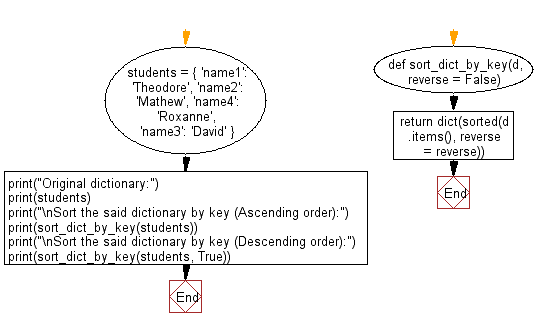﻿ Python: Sort a dictionary by key - w3resource# Python: Sort a dictionary by key

## Python dictionary: Exercise-14 with Solution

Write a Python program to sort a given dictionary by key

Sample Solution-1:

Python Code:

``````color_dict = {'red':'#FF0000',
'green':'#008000',
'black':'#000000',
'white':'#FFFFFF'}

for key in sorted(color_dict):
print("%s: %s" % (key, color_dict[key]))
```
```

Sample Output:

```black: #000000
green: #008000
red: #FF0000
white: #FFFFFF
```

## Visualize Python code execution:

The following tool visualize what the computer is doing step-by-step as it executes the said program:

Sample Solution-2:

• Use dict.items() to get a list of tuple pairs from d and sort it using sorted().
• Use dict() to convert the sorted list back to a dictionary.
• Use the reverse parameter in sorted() to sort the dictionary in reverse order, based on the second argument.

Python Code:

``````def sort_dict_by_key(d, reverse = False):
return dict(sorted(d.items(), reverse = reverse))
students = { 'name1': 'Theodore', 'name2': 'Mathew', 'name4': 'Roxanne', 'name3': 'David' }
print("Original dictionary:")
print(students)
print("\nSort the said dictionary by key (Ascending order):")
print(sort_dict_by_key(students))
print("\nSort the said dictionary by key (Descending order):")
print(sort_dict_by_key(students, True))
```
```

Sample Output:

```Original dictionary:
{'name1': 'Theodore', 'name2': 'Mathew', 'name4': 'Roxanne', 'name3': 'David'}

Sort the said dictionary by key (Ascending order):
{'name1': 'Theodore', 'name2': 'Mathew', 'name3': 'David', 'name4': 'Roxanne'}

Sort the said dictionary by key (Descending order):
{'name4': 'Roxanne', 'name3': 'David', 'name2': 'Mathew', 'name1': 'Theodore'}
```

Flowchart:## Visualize Python code execution:

The following tool visualize what the computer is doing step-by-step as it executes the said program:

Python Code Editor:

Have another way to solve this solution? Contribute your code (and comments) through Disqus.

What is the difficulty level of this exercise?

Test your Programming skills with w3resource's quiz.

﻿

## Python: Tips of the Day

Decapitalizes the first letter of a string:

Example:

```def tips_decapitalize(s, upper_rest=False):
return s[:1].lower() + (s[1:].upper() if upper_rest else s[1:])
print(tips_decapitalize('PythonTips'))
print(tips_decapitalize('PythonTips', True))
```

Output:

```pythonTips
pYTHONTIPS
```